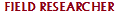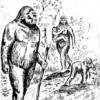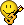# Thinker Thunker size comparison of Patty

## Recommended PostsPhotogrammetry 101

90% of photogrammetry is solving triangles. Since we lose the 3rd dimension on film we only have FOV (field of view) angles to work with. The object is then to derive the sides of the triangles which represent distances from the camera.

The vertical and horizontal angles of view on a full frame of film depends on the focal length of the lens and the dimensions of the camera's aperture gate, which restricts the field of view from circular to rectangular. Here is the K100 full FOV and the rectangular gate that represents a full frame of film:The horizontal angle of view for the PGF (25mm) = 23.26 degrees.

Here is the Camera-T1-T2 triangle relative to the HAV and the 3 rules for solving triangles.

Note that the Tree triangle calcs the distance between them at 14 feet, which is right in the ballpark of the measured distance between them of 12 feet. Also note that the distance between T1 and T2 was likely measured from the outside circumference of each tree at breast height and not the center of each tree at ground level. That could have underestimated the distance by 1 to 2 feet and puts the photogrammetry in sync with the measured distances and confirms that a 25mm lens is most probable. Smaller focal lengths only increase the distance between the trees.

The angles between any 2 objects on the film can be measured but unless we know at least 3 of the six triangle measurements (A,B,C,a,b,c), we can't derive the other three to solve the triangle. Refer to the 3 triangle formulas.

•1
•3

##### Share on other sitesNice work with the image of the K100 viewfinder lens.  I believe I have seen it posted here on the BFF years ago. The 3 crosses are aiming aids that Kodak created. There is a separation distance between the taking lens and viewing lens so there is a compensation factor to center the target. The larger cross at the top middle is for targeting items that are further than 7'.  The middle and lower crosses are for targeting very close subjects, less than 7' away. The  K100 camera series is not a 'run and gun' system. Try looking through that 25mm viewfinder and run through a forest, creek bed, whatever.  Roger did well moving on that terrain.

•1

##### Share on other sitesNice work, Giganto.

I have one question....you gave the 'HAV' for the PGF camera as "26.23" degrees....but on the protractor, it looks like the HAV is about 42 degrees.

I don't understand why there is a difference between those two figures. Could you please explain??I have recently done some work, along this very line...and produced a couple of graphics showing how much of the far hillside would have been captured by a 25MM lens on the camera....(at the supposed distance from the hillside, for the camera/Roger)….but I'd like to get a better understanding of the HAV before posting my graphics.

##### Share on other sitesBFF Patron

^ actually Giganto. gave 23.26 deg. in his above reference not 26.23,  just saying, question still applies and a good one at that

##### Share on other sites8 hours ago, SweatyYeti said:

Nice work, Giganto.

I have one question....you gave the 'HAV' for the PGF camera as "26.23" degrees....but on the protractor, it looks like the HAV is about 42 degrees.

I don't understand why there is a difference between those two figures. Could you please explain??I have recently done some work, along this very line...and produced a couple of graphics showing how much of the far hillside would have been captured by a 25MM lens on the camera....(at the supposed distance from the hillside, for the camera/Roger)….but I'd like to get a better understanding of the HAV before posting my graphics.

Right you are SY. This is what peer review is all about. I was using an old graphic where I was calculating the HAV for a wide angle lens and I mistakenly assumed it was for a 25mm lens. Also, my intent was to show how to solve triangles based on any size lens and an example using a 25mm lens put the protractor too far from the image to measure such small angles.

However, we don't actually need the protractor which is why I introduced the triangle formulas. Here is the tree triangle based on a 25mm lens:

Full frame = 1683 pixels HAV = 23.26 degrees

T1->T2 = 228 pixels HAV = 228/1683 x 23.26 = 3.2 degrees

Using the cosine rule:Historical distances to T1 = 85 feet,  T2 = 95 feet

Distance between T1 and T2 = b = sqrt(95*95+85*85-2*95*85*cos(3.2)) = 11.2 feet

SY thinks ( as do I) we should add 20 feet to the distances  to T1 and T2:

b = sqrt(115*115+105*105-2*115*105*cos(3.2)) = 11.7 feet

If SY is correct and Roger's camera position was 20 feet farther back than historical claims, then the distance between T1 and T2 based on a 25mm lens is 12 feet

We have a bingo!

Edited by Gigantofootecus
•1

##### Share on other sites7 hours ago, Gigantofootecus said:

Right you are SY. This is what peer review is all about. I was using an old graphic where I was calculating the HAV for a wide angle lens and I mistakenly assumed it was for a 25mm lens. Also, my intent was to show how to solve triangles based on any size lens and an example using a 25mm lens put the protractor too far from the image to measure such small angles.

However, we don't actually need the protractor which is why I introduced the triangle formulas. Here is the tree triangle based on a 25mm lens:

Full frame = 1683 pixels HAV = 23.26 degrees

T1->T2 = 228 pixels HAV = 228/1683 x 23.26 = 3.2 degrees

Using the cosine rule:

Thanks for the clarification/correction, Giganto.That will help me to work with the Triangle formula.

Quote

Historical distances to T1 = 85 feet,  T2 = 95 feet

Distance between T1 and T2 = b = sqrt(95*95+85*85-2*95*85*cos(3.2)) = 11.2 feet

SY thinks ( as do I) we should add 20 feet to the distances  to T1 and T2:

b = sqrt(115*115+105*105-2*115*105*cos(3.2)) = 11.7 feet

If SY is correct and Roger's camera position was 20 feet farther back than historical claims, then the distance between T1 and T2 based on a 25mm lens is 12 feet

We have a bingo!

Those numbers all seem to make sense.  The distance between Trees T1 and T2 must have been 12 feet....that was the distance measured by both John Green, and Rene Dahinden.

On a related note....here is a graphic I recently put together....using Steven Streufert's Filmsite Diagram...(which was drawn to scale, with each grid square equal to 10' by 10')….to try to calculate the distance across the far hillside that would be captured by a 25MM lens...

As a result of Bill Munns' recent post, in which he posted the cross-field measurement made of the distance between the 'Big Tree' and the "Hardy" tree....I  just added the bottom two squares to the graphic:

The 1st square:  the distance from the 'Big Tree' to the "Hardy Tree", as recently measured at the filmsite....(82 feet.)

The 2nd square:  the distance between those two trees, as measured in my graphic....using the number of pixels between the two trees, divided by the 'pixel/inch' figure...of .15 p/in....(87 ft.)

Those two figures are pretty close to each other...and my graphic is not super-precise. So, there could potentially be a closer match in those distances....by a simple adjustment of the 'distance from the camera, to the far hillside'.

Edited by SweatyYeti

##### Share on other sitesOn 9/25/2019 at 12:40 PM, Gigantofootecus said:

This involves ortho-rectification, which requires a lot of algorithms, which you just have to take at face value.

I knew ortho-rectification was the key!(just joking, I had no idea what that is so; I googled it)

"Orthorectification is the process of removing the effects of image perspective (tilt) and relief (terrain) effects for the purpose of creating a planimetrically correct image."  That is definitely a very important, albeit fairly complicated, process required for solving PGf image details.

It's great to see you back and posting real Science again here, Gigantofootecus!  thank-you  8 )##### Share on other sites"Turning the tables" on ThinkaThunka's "Proof" that Patty was about 7 1/2' tall....(crouched down)…..I have Proof that TT is only about 3 feet tall...

…..courtesy of his "18 inch" wide trees...TC-1 and TC-2.

In actual fact...it is much more likely that those trees were in the 8 to 9 inch range, in diameter.